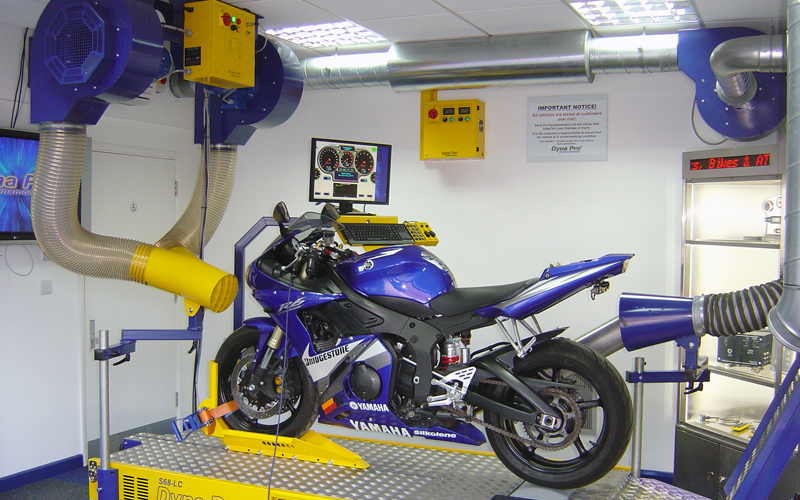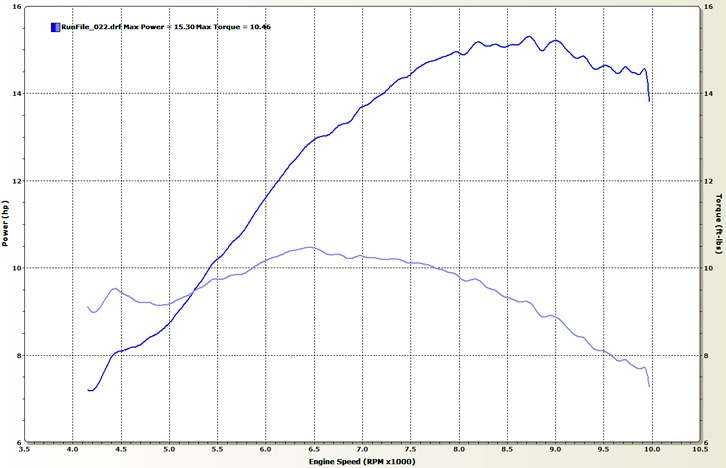# Moto-Tech – The dynamo meter

PAR Michel Garneau Posted on

### Who’s got the power?

Engine power has been a major preoccupation of motorsport fans for a long time. Indeed, discussions between enthusiasts of motorcycles, cars or other vehicles often focus, unsurprisingly, on power. In last month’s column, we tackled the fundamentals of engine power. This month, we will jump from the theoretical to the practical realms by delving into an essential tool used to measure the torque and power of an engine package, the dynamometer, more commonly called simply “the dyno.”

Power: From Watt to today

As we discussed in the last issue, horsepower is a measure of an engine’s ability to do work (within a certain amount of time) and this depends on two elements, force (torque) and speed. In truth, there is no way to directly measure an engine’s power. However, all is not lost as we have the means to measure the two aforementioned critical elements, allowing us to arrive at it indirectly by simple math.

Various units of measure exist for measuring power, the most common of which (at least in North America) is horsepower. Originally, this unit of measure, invented by British engineer James Watt (at the beginning of the Industrial Revolution), was defined as the ability to lift a weight of 33,000 pounds a distance of one foot in one minute, approximating the work capacity of a horse. Things have evolved since and an equivalent formula has been developed (a method that is, quite obviously, simpler to use than Watt’s) :

power in hp = torque (in lb-ft) x speed (rpm) ÷ 5252

Notice how the formula includes the two factors mentioned above (torque and speed).

Other units of measure for power include the Watt (W or kiloWatt) and metric horsepower (mhp), the latter sometimes leading to confusion (especially when the m is deleted). For the record, one mhp is equal to 98.6% of an Imperial hp (in other words, 100 mhp is equal to 98.6 hp). It is worth noting, somewhat unsurprisingly, that European manufacturers have a tendency to use the Metric unit, which tends to inflate their claimed power numbers slightly.

The dynamometer at work

The dynamometer is a device, a tool, that we hook up to the engine, either directly or indirectly, that allows us to measure engine torque and speed. As demonstrated previously, with this data in hand, we can then calculate engine power. Different types of dynos exist but these generally fall into one of two categories: inertia or brake type.

In the case of inertia dynos (by far the most common type used in the motorcycle industry), the dynamometer requires that the engine (or the rear wheel to be precise) spin a heavy drum, torque being calculated from the latter’s acceleration. In the case of the brake dyno, the unit applies a resistance to the engine (i.e., “braking” it) and torque is calculated from the amount of effort required to slow down the engine. Assorted braking systems are used, one of which is a water recirculation configuration, consisting of fluid being pumped though some piping. As the engine runs, an adjustable valve is slowly closed to restrict the movement of the fluid while the power is calculated by measuring the flow volume, fluid pressure, and engine speed. Another type from this family is the “Eddy current” dyno, which operates on the premise of forcing a conductive material to rotate through an electromagnetic field, the reverse force created by the resulting Eddy current acting to resist engine torque. In all instances, computers use complex formulas to calculate torque across the engine’s operating speed range. This information is then combined with engine speed data to calculate engine power.

It goes without saying that power readings obtained directly at the engine’s crankshaft are superior to those measured at the rear wheel, namely because crankshaft numbers are not compromised by drivetrain friction and slippage.Dynamometer results are often displayed in table format, or as a graph, as seen here. In order to obtain the “torque curve,” measures obtained at various engine speeds are linked together. Insofar as the power curve is concerned, the data points are obtained using the torque measures and respective engine speed readings. Note how the two curves (torque in purple and power in blue) cross at exactly 5252 rpm.